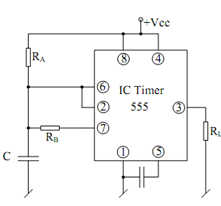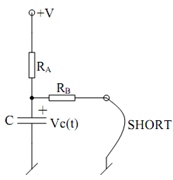## Alternative Astable Multivibrator Assignment Help

Assignment Help: >> IC Timer - Alternative Astable Multivibrator

Alternative Astable Multivibrator with 50% Duty Cycle:

In yet another scheme, the diode is ignored altogether and still one may obtained 50% duty cycle.Figure: Alternative Astable Multivibrator with 50% Duty Cycle

As with the earlier circuit, while V03 is high, pin 7 is open and the charging of the capacitor takes place through RAC therefore, TH remains TH  = RA C ln 2.

For the duration for which V03 being Low and pin 7 acting as a short, the time TL may be calculated as follows. The discharge equivalent circuit may be made as follows :Figure: Equivalent Circuit for the Case when Pin 7 Acts as a Short

Before the situation represent in the equivalent circuit arises, the following apply :

at t = 0,  V c  (0) = (2/3) V cc